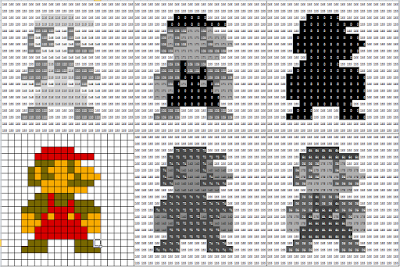2017年1月24日

段考進步怎麼看(2)-利用python製作學期成績PR變化折線圖=====以下是python3的code＝＝＝＝＝
# -*- coding: utf-8 -*-
#coding:utf-8

"""
Created on Wed Jan 18 21:41:17 2017

@author: pancala
"""
#讀取csv檔
import os
import pandas as pd
os.chdir("/media/pancala/3T/試題分析/105")
file='105A.csv'

#定義中文，字型檔用  fc-list :lang=zh 查詢
from matplotlib.font_manager import *
myfont = FontProperties(fname='/usr/share/fonts/opentype/noto/NotoSansCJK-Regular.ttc')
#解決負號'-'顯示為方塊的問題
matplotlib.rcParams['axes.unicode_minus']=False

import numpy as np
import matplotlib.pyplot as plt

#把任教班級取出
for stuclass in [7,8,16,18]:
#for stuclass in :
#篩選出特定班級
filter = data["班級"] == stuclass
new_data = data[filter]

#計算班級人數
studentNum = new_data.shape

#subplot的列數由總班級人數決定
subplotRowNum = int(np.ceil(studentNum/5))

#設定subplots的列數、欄數和大小
fig, axarr = plt.subplots(nrows=subplotRowNum,
ncols=5,
sharex=False,
sharey=False,
figsize=(10,subplotRowNum*2+2))

#設定主標題
fig.suptitle(str(stuclass)+ "班" +"上學期生物段考PR變化",fontproperties=myfont, size=20)
#plt.title(str(stuclass)+ "班" +"上學期生物段考PR變化",fontproperties=myfont, fontsize=10)

#各小圖之間的間隔設定

#把每一個學生的資料取出
for i in range(0,studentNum):
#for i in :
#座號
num = new_data.iloc[i,3]
#座號前面補零
if num <10:
num=str(0)+str(num)

#姓名
name = new_data.iloc[i,4]
#A1的PR
a1 = new_data.iloc[i,8]
a1 = float(a1)
#A2的PR
a2 = new_data.iloc[i,9]
a2 = float(a2)
#A3的PR
a3 = new_data.iloc[i,10]
a3 = float(a3)

x= [1,2,3]
y = [a1,a2,a3]

#畫出PR折線圖
axarr[int(i/5),i%5].plot(x,y)

#限定y軸大小
axarr[int(i/5),i%5].set_ylim([-5,105])

#設定小圖的標題為姓名
axarr[int(i/5),i%5].set_title(str(num) + name,fontproperties=myfont,size=15)

#X軸標示設定為一二三
axarr[int(i/5),i%5].set_xticks((1,2,3))
axarr[int(i/5),i%5].set_xticklabels( ("一","二","三"),fontproperties=myfont)
axarr[int(i/5),i%5].tick_params(labelsize=10)

#plt.show()

#班級前面補零
if stuclass <10:
stuclass=str(0)+str(stuclass)
else:
stuclass=str(stuclass)

fig.savefig(stuclass + ".png",dpi = 250)
#plt.close()

2017年1月20日

大手攜小手的數理科學營-手作顯微鏡與行動顯微鏡課程2017年1月16日

手機app研究擴大機的聲音

Physics Toolbox Sensor Suite

Spectrogram

2017年1月5日

用Excel VBA理解像素的數字和顏色（拆解的方式，是用ImageJ的功能，去分解，然後儲存成文字檔）